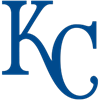Loading AL Championship Futures...DraftKings AL Championship Odds
+240
2.4 to 1Yankees
23.0% implied probability

+400
4 to 1White Sox
15.7% implied probability

+700
7 to 1Twins
9.8% implied probability

+750
7.5 to 1Astros
9.2% implied probability

+850
8.5 to 1Rays
8.2% implied probability

+875
8.75 to 1Blue Jays
8.0% implied probability

+1100
11 to 1Athletics
6.5% implied probability

+1700
17 to 1Angels
4.3% implied probability

+2000
20 to 1Indians
3.7% implied probability

+2300
23 to 1Red Sox
3.3% implied probability

+4000
40 to 1Orioles
1.9% implied probability

No changes have been recorded yet.
+4000
40 to 1Royals
1.9% implied probability

+5000
50 to 1Mariners
1.5% implied probability

No changes have been recorded yet.
+5000
50 to 1Rangers
1.5% implied probability

No changes have been recorded yet.
+6000
60 to 1Tigers
1.3% implied probability

No changes have been recorded yet.